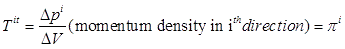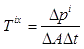## Pages

### 13. Stress-Energy-Momentum Tensor

In this section we will now pursue the goal of understanding how the field equation of Einstein is obtained.

The Einstein’s field equation consists of two crucial concepts, one of them being the geometry of the curved spacetime and the other being the cause of this curved spacetime. Einstein believed that this curvature of spacetime was due to the presence of mass or in other words, “The effect of the mass-energy creates a curvature in the spacetime.”

We have discussed the geometric part (curved spacetime) of the equation in the above mathematical section; however we have not discussed the idea of the momentum-energy tensor (which is the source of the curvature).

While we may be tempting to answer the question about why mass effects spacetime, we have to understand that, it isn’t an easy problem. However, this does not stop us from assuming that mass does curve the spacetime. We in fact use the assumption that the curvature of spacetime reduces to the Newtonian approximation in the limits of the weak field and low density without any known mechanism for the curvature itself.

What then is an Energy-Momentum tensor?

Let us consider a four-vector $p^{\alpha}$. The components of this four-vector momentum are the energy and the three momentum vectors. The question that is important here is, how can we associate four-vector $\Delta p^{\alpha}$ with the three volume $n_{\beta} \Delta V?$. The answer for this question is a tensor of an object with two indices $T^{\alpha\beta}$. This object is called the energy-momentum Tensor. We can write down the relationship as follows,
To understand what the components of the energy-momentum tensor are, consider a particular inertial frame in flat spacetime and a three-dimensional volume $\Delta$ at rest in that frame. That volume is part of a t = constant. We can choose the normal of the three-surface in spacetime to be $n_{\alpha}=(1,0,0,0)$. With this choice of normal equation $(1)$ becomes,$(2)$
Now from $(2)$ it follows that,Thus we understand the significance of four of the components of the stress-energy tensor. Similarly if we consider a time like three volume spanned by intervals $\Delta y, \Delta z, \Delta t$ then, the unit normal to this three surface pointing in the x-direction is $n_{\alpha}=(0,1,0,0)$. The analog is then,

The time component of this equation gives,The spatial part can be written as follows,Now this can be written in general force term as,
Thus, $T^{ij}$= $i^{th}$ component of the force per unit area exerted across a surface with normal in direction $j$

In classical mechanics a force per unit area is called a stress, and $T^{ij}$ is called the stress tensor. In special case when $(i=j)$, we have the usual pressure components. All in all we can summarize the $T^{\alpha\beta}$ as the following,
Where, $\varepsilon ,\pi ^{i},T^{\alpha\beta}$ are the energy density, momentum density and the stress tensor respectively.